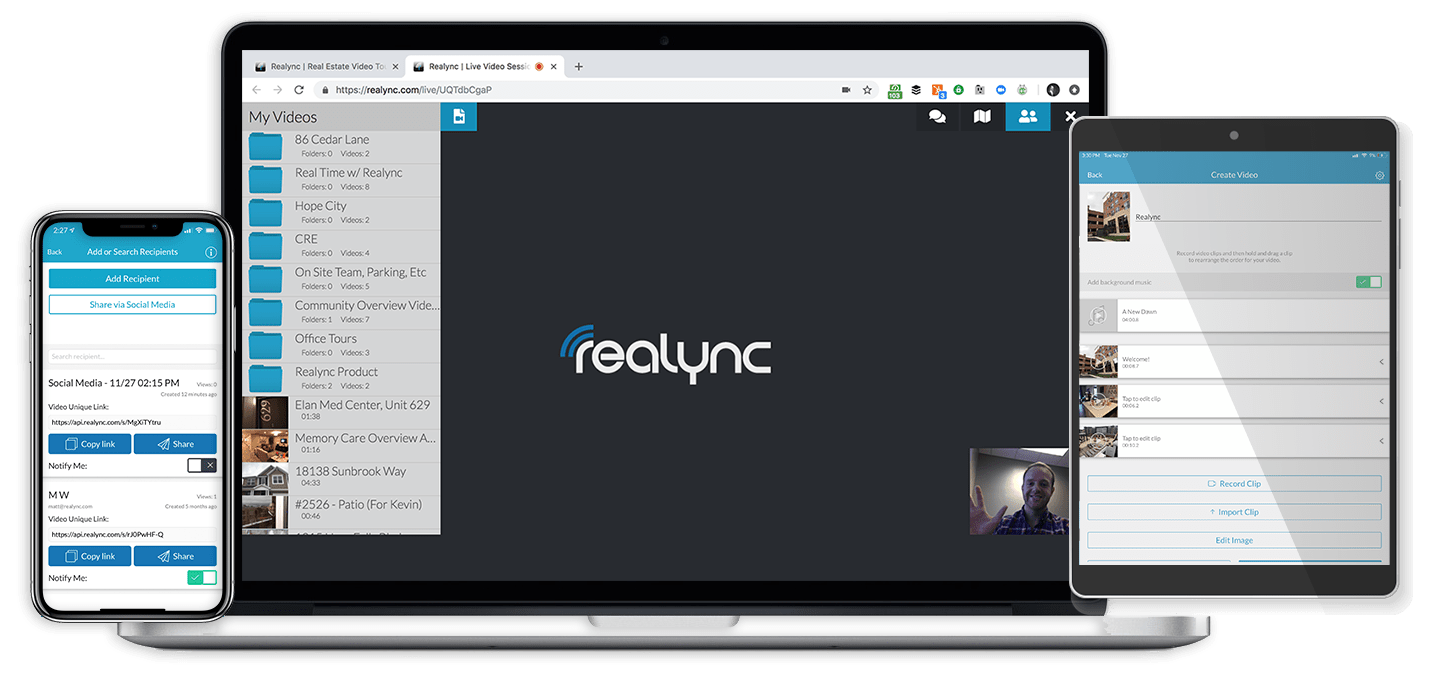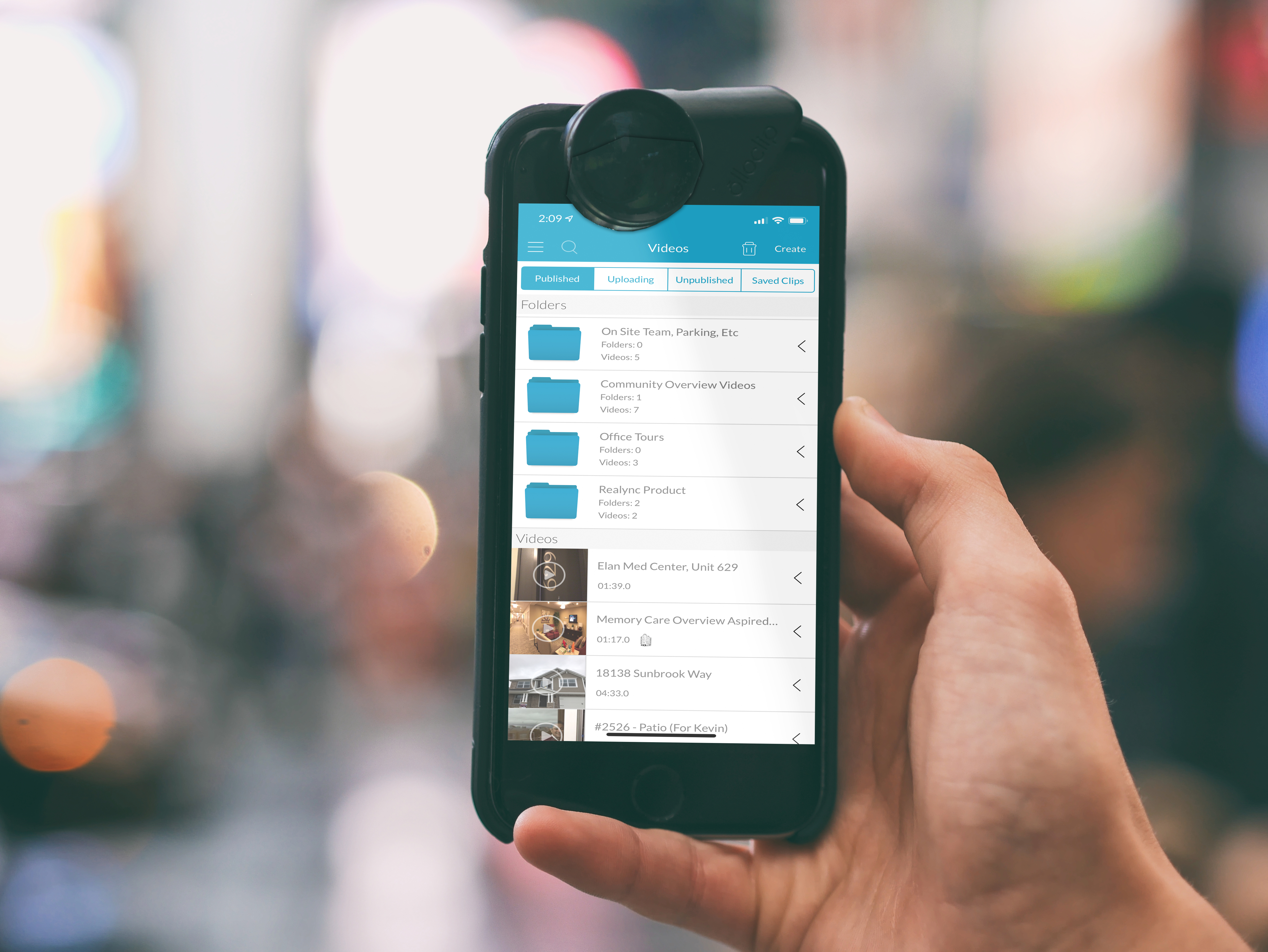## Close More Site-Unseen LeasesStart converting more leads and engaging residents better today.

# Apartment Occupancy Calculator

The apartment occupancy rate is the ratio of rented units or gross income as compared to the maximum potential. It can be calculated as physical occupancy or economic occupancy. It can be calculated at one point in time and can also be examined over time to detect trends. Knowing the occupancy rate is important for an apartment building investor for several reasons. The first is that it helps identify anticipated cash flow. It also can help you gauge how well the apartments are being managed. If the building you have invested in or anticipate investing in has a low occupancy rate relative to others around it, this may indicate that the building is in need of sprucing up, new marketing materials or better management. If the occupancy rate is higher than comparable apartment buildings nearby, this may indicate a well-managed and well-maintained property.

An apartment occupancy calculator can make using this formula easier. It also can help you develop models to track when leases may come up for renewal and which ones might be due for a rate increase. This will improve long-term profitability in your investment.

How Can an Apartment Occupancy Calculator Help Save You Time and Money?## Apartment Occupancy Rates### Regularly benchmarking the apartment occupancy rates of your apartment buildings against that of others in the area and country is important.

This helps you answer the question what is a good occupancy rate for an apartment building. Once you answer this question, you can determine how well your building is performing. In 2019, a good occupancy rate against a national average is 96 percent or more. However, apartment occupancy rates do vary depending upon locality.

The U.S multifamily vacancy rate reached 8 percent in the second quarter of 2020, up from four percent in the previous quarter, according to The effects of the COVID-19 pandemic has led the growth in building of more apartment buildings to slow.

Performance in the multifamily market was solid through the end of 2019 and into the first quarter of 2020, with stable occupancy and moderate rent growth. However, investment activity has declined through the second quarter of 2020 likely due to effects of the COVID-19 pandemic, according toThe future is now and with our captions and translations, you can reach out to any audience, anywhere in the world. Every experience you create is saved to the cloud instantly so you can have the peace of mind that comes with knowing that you’ll always have access to the materials you require.## Calculate Occupancy Rate### Physical

Physical occupancy rate is the percentage of The occupancy rate formula for a particular month is number of units rented/ number available to be rented* 100. For example, you may have 50 units available for renting and 45 of them have paying tenants. To calculate physical occupancy rate, divide 45 by 50 for a total of .90. To express as a percentage multiply .90 by 100. The physical occupancy rate is 90 percent.

Some people confuse physical occupancy and leased occupancy. Here’s how to calculate the leased percentage: current number of units occupied + (number of units with signed leases yet to move in) / total number of units * 100%. This measurement can be misleading. For example, you may have units occupied with people moving out next month but also signed leases for people moving in next month. This would result is you showing an occupancy of more than 100 percent.

### Economic

An economic occupancy rate is the proportion of the gross potential rent that is collected—the money that is actually paid to your business. The gross potential rent (GPR) is the amount of rent that would be collected if you rented out all the apartments at the amount of rent shown in the rental contract. The economic occupancy rate formula is (GPR minus deductions)/GPR * 100. To calculate the economic occupancy apartment building owners first calculate the GPR by multiplying the number of apartments by the amount of rent that you could charge for each; for example, 70 apartments at \$200 each would yield a GPR of \$14,000. Then deduct out the rent not received because units are vacant or occupied by someone who receives rent for free, such as the building supervisor, and the amount of discounts given. Let’s say that one apartment is vacant and one is occupied by a supervisor. Another apartment is discounted \$100. That means you would deduct \$500 from the \$14000 to get a total of \$13500. Divide \$13500 by \$14000 and multiply that by 100. Your economic occupancy rate is 96.4 percent.

### Room

A room occupancy calculator is important to ensure that you comply with state laws regarding how many people can live in an apartment unit. The room occupancy formula is total square feet / number of tenants occupying the apartment. For example, New York law says that you must have at least 80 square feet per person. Suppose you have three people living in a two-room apartment. One room is 8×10 (80 square feet) and the other room is 12×14 (168 square feet). The total square footage is 248 (80 plus 168) Divide 248 by the number of tenants (3) to get 82.6. From this you determine that you have 82.6 square feet per person and are in compliance with the law.The real estate industry was once reliant on photos and stale, overpriced videos. In a climate where the buyer is going to make a more educated decision than ever before, Realync provides the tools necessary to create the authentic experience today’s market is looking for.## Occupancy Forecast Formula

While knowing where you stand with occupancy at any given point in time is important, knowing where you are going is also important for developing a plan of how to increase apartment occupancy rates — both physical and economic — over time. To build an accurate forecast, you must first understand your occupancy patterns over time. This means keeping track of several months or years of data, perhaps on an Excel spreadsheet or in your property management software. In addition to historical physical and economic occupancy rate data, you would also keep track of when each lease will expire so that you can see in which months you potentially will have a vacancy and act early to prevent a vacancy. Keeping track of the amount of rent paid for each unit, as well as researching the amount of rent charged for comparable apartments in your area, will let you know which apartments might merit a rate increase. Since rents and physical occupancy in one apartment building are affected by the supply of similar housing in the same neighborhood, you would keep track of how much building is being done in your area, as well.## How to Calculate the Occupancy Rate in Excel

Excel is a good tool for calculating occupancy rates each month and over time. One example of how to calculate the occupancy rate in excel is to create a spreadsheet with pages for each month. You then enter the monthly data along with the occupancy formula in excel. An example of one page of the spreadsheet is below. In this example, which calculates economic occupancy, the maximum rent is listed for each unit in column B and a formula in the bottom row of column B totals the maximum rent. The current rent is listed in column c, with a formula in the bottom row to total that. Then the economic occupancy formula, in this case, c9/b9, is in the bottom row of column D. You change the formatting of the cell in excel to have this expressed as a percentage.

Unit NumberMaximum RentCurrent RentEconomic OccupancyLease Expires
1\$200.00\$150.00March
2\$400.00\$400.00April
3\$200.00\$0.00
Totals\$800.00\$550.0068.75%## Vacancy Rate Calculator

The vacancy rate is the opposite of the physical occupancy rate. The rental vacancy rate formula is 100 minus the occupancy rate. For example, if your occupancy rate is 95 percent, the vacancy rate is 100 minus 95 or 5 percent. To benchmark your performance, compare your vacancy rate to the rental vacancy rate by city and state. For an even more accurate benchmark, compare with the rental vacancy rate by zip code. Related to the vacancy rate is the availability rate, which is the ratio of available space to total rentable space, calculated by dividing the total available square feet by the total rentable square feet.## Double Occupancy Rate

The double occupancy rate refers to those apartments that are occupied by two adults.

The double occupancy formula is the number of apartments occupied by two adults/number of occupied apartments and multiplied by 100 to obtain a percentage. This number will typically be smaller than the physical occupancy rate because some apartments will be occupied by only one person. Similarly, the multiple occupancy percentage formula is number of apartments occupied by more than one adult/number of occupied apartments and multiplied by 100 to obtain a percentage.## Double Occupancy Rate

The double occupancy rate refers to those apartments that are occupied by two adults.

The double occupancy formula is the number of apartments occupied by two adults/number of occupied apartments and multiplied by 100 to obtain a percentage. This number will typically be smaller than the physical occupancy rate because some apartments will be occupied by only one person. Similarly, the multiple occupancy percentage formula is number of apartments occupied by more than one adult/number of occupied apartments and multiplied by 100 to obtain a percentage.## Related Calculations and Formulas

Several other calculations are important to understanding how your multifamily apartment building is performing compared with similar properties.

• The average daily rate is calculated using this formula: Revenues earned from units/Number of units rented. A rising average daily rate means that your investment is becoming more profitable
• Revpar is revenue per available unit. The revpar formula is average daily rate * physical occupancy rate.
• Loss to lease refers to money lost through making incentives to perspective tenants, such as one month’s free rent with the signing of a one-year lease.